Finding Inverse

Chapter 1 Class 12 Relation and Functions
Concept wise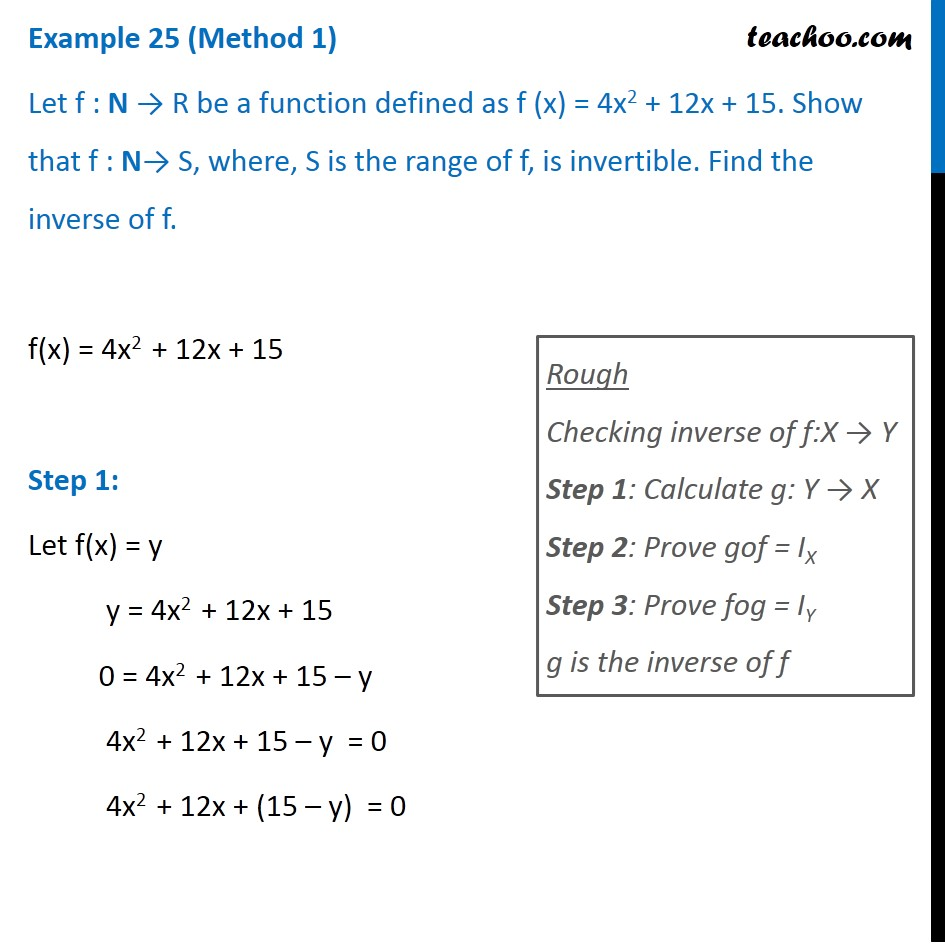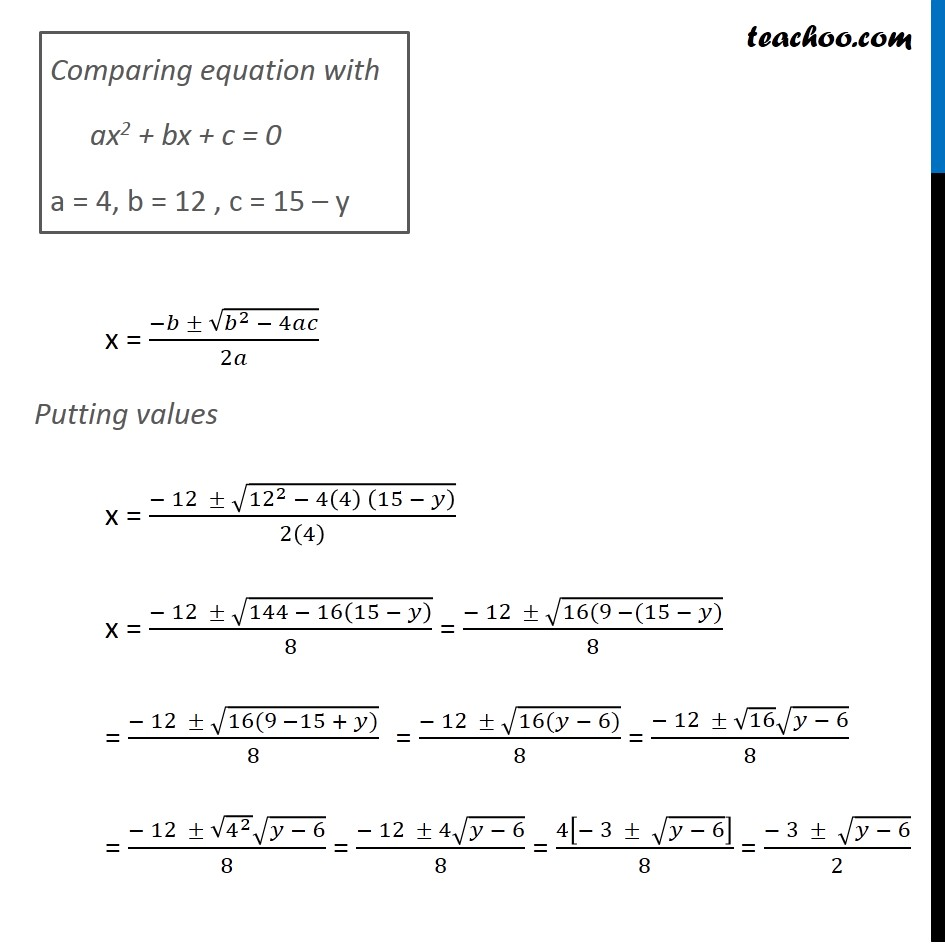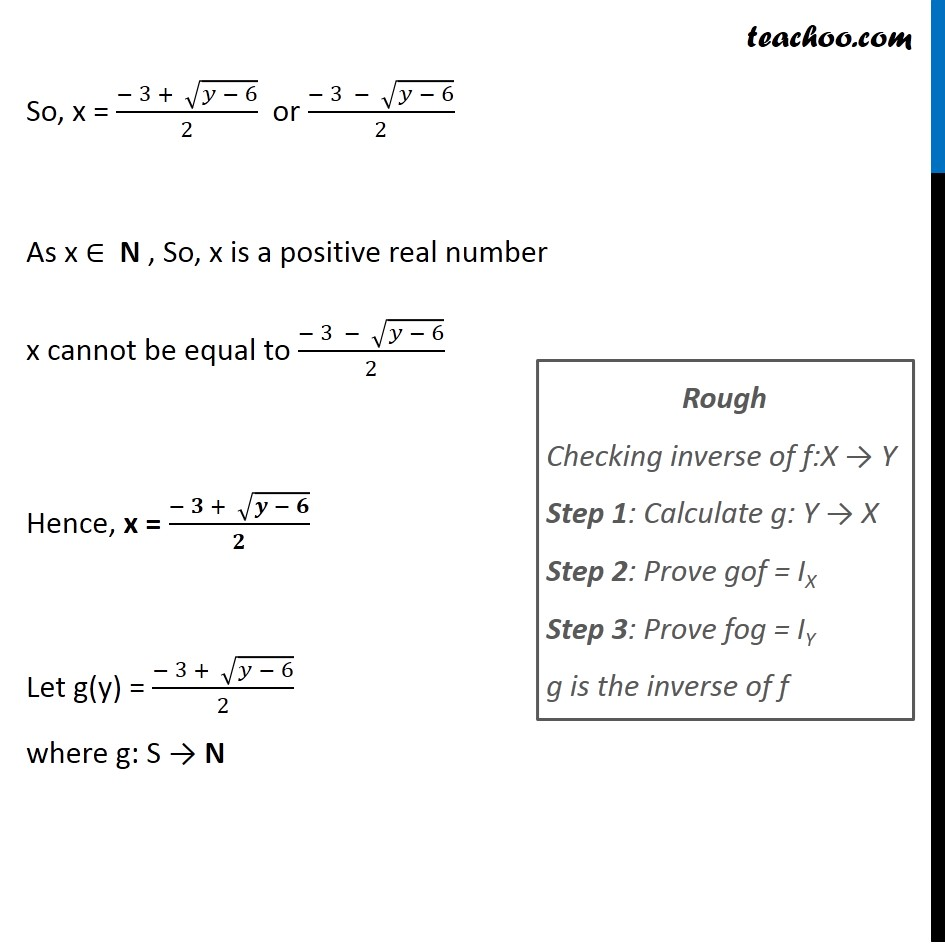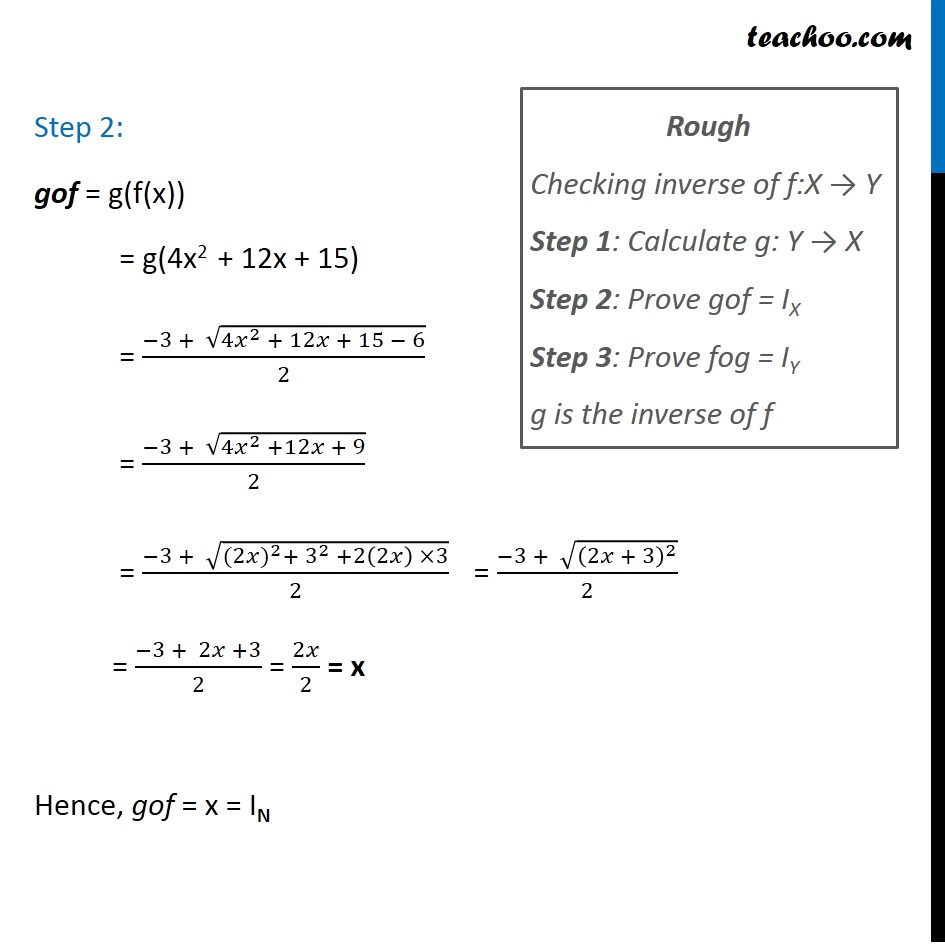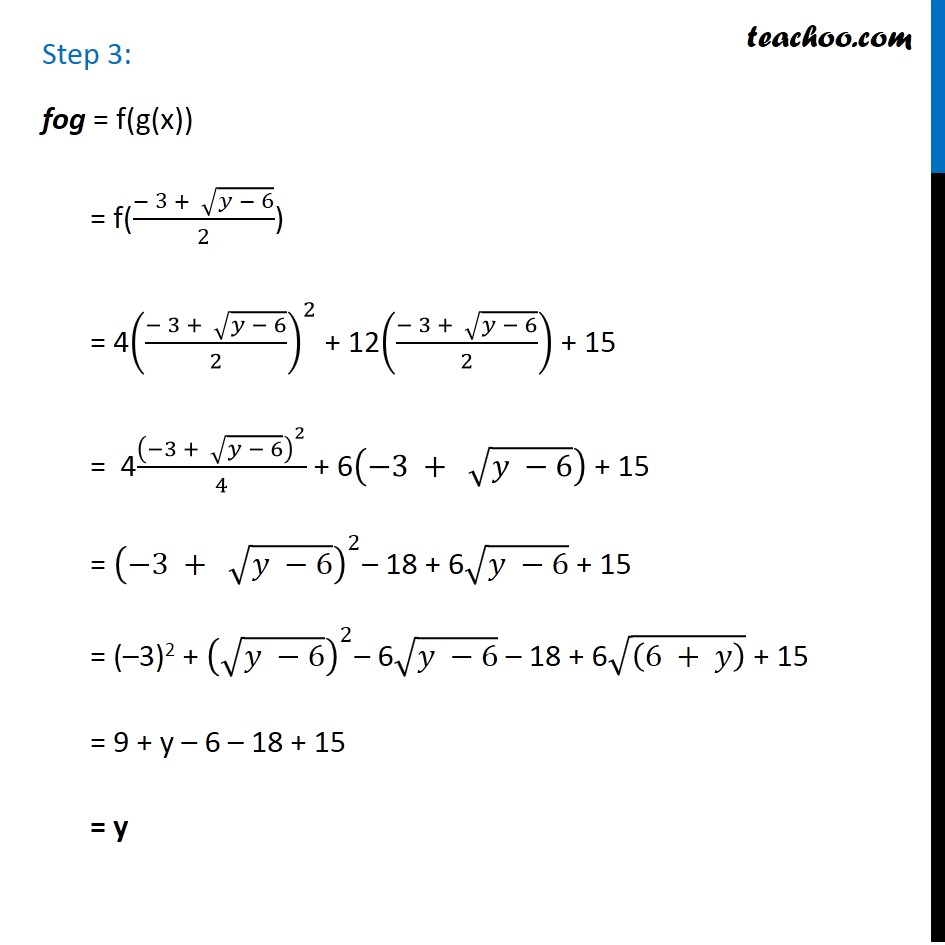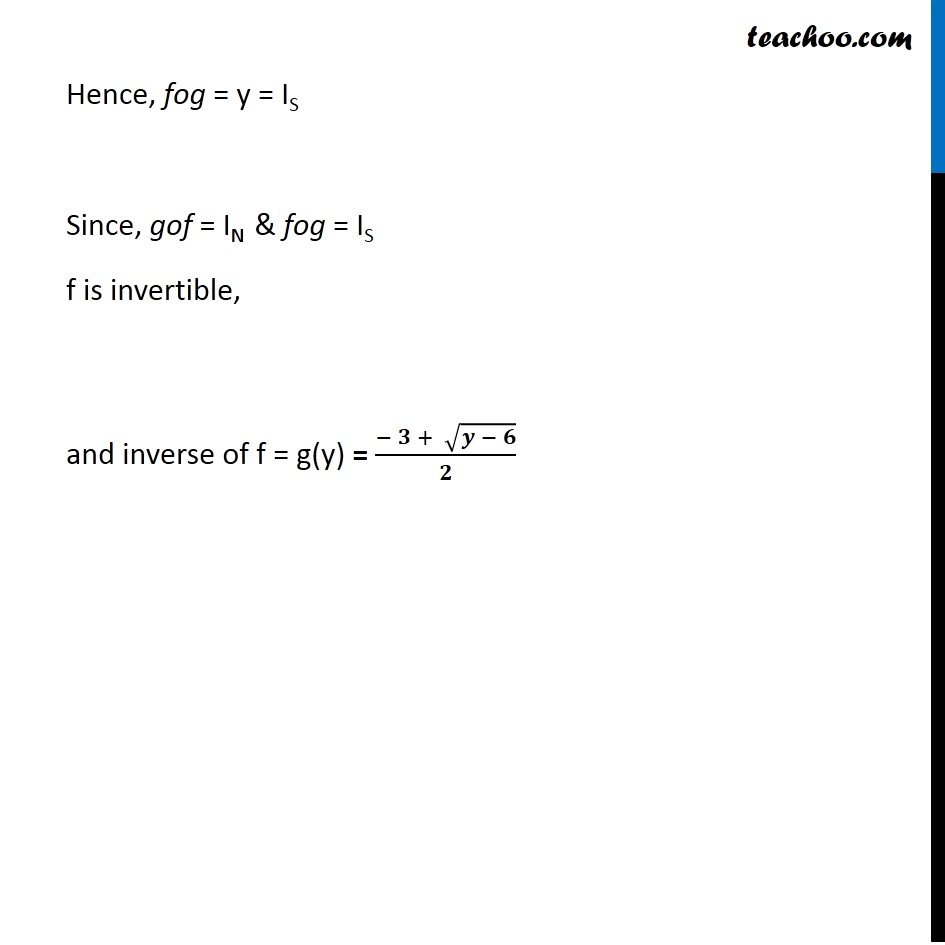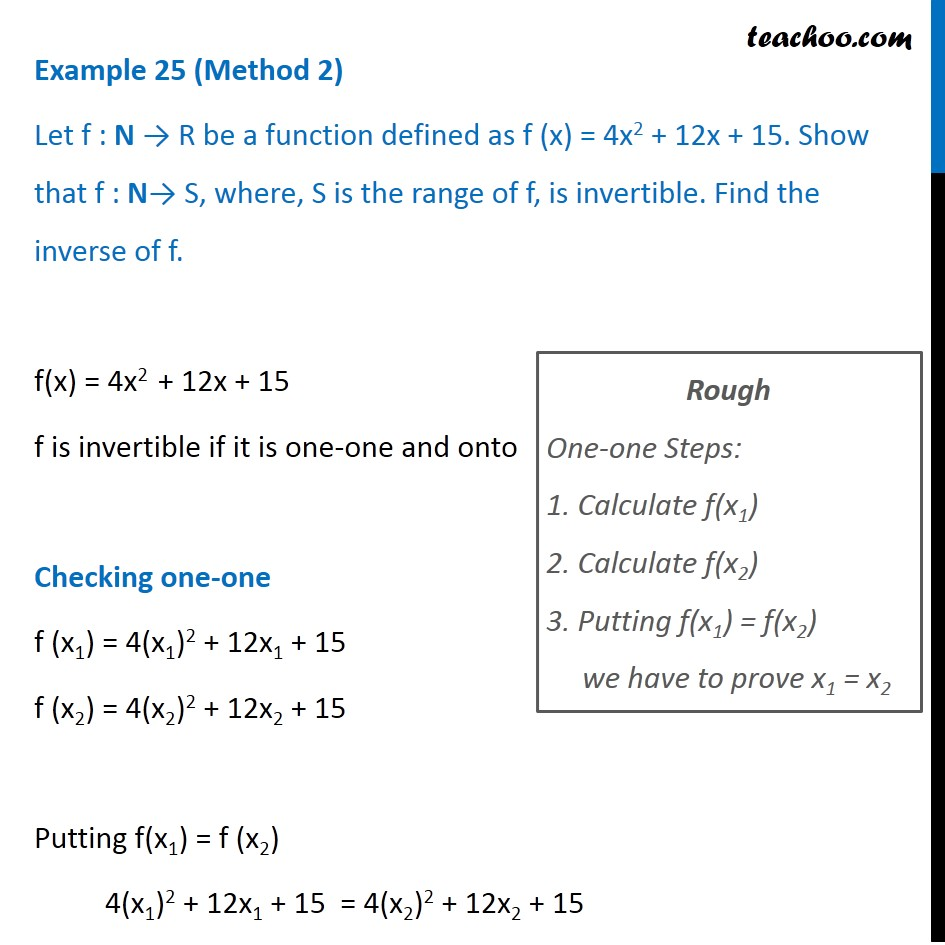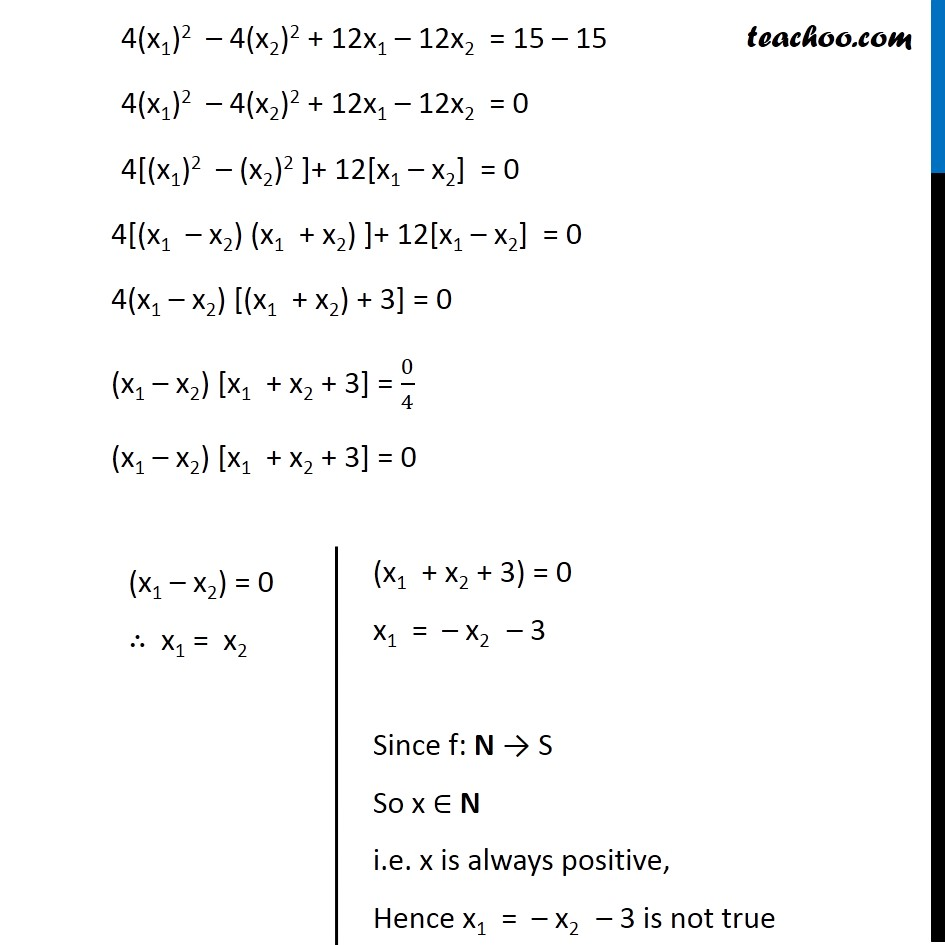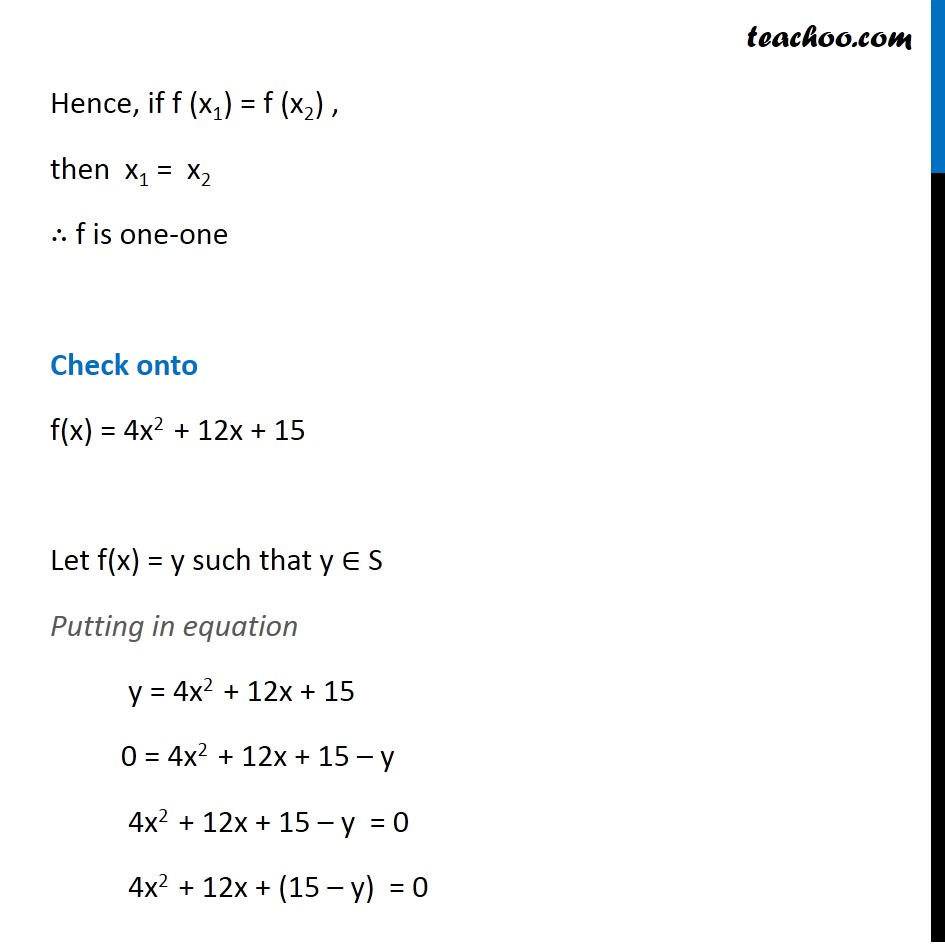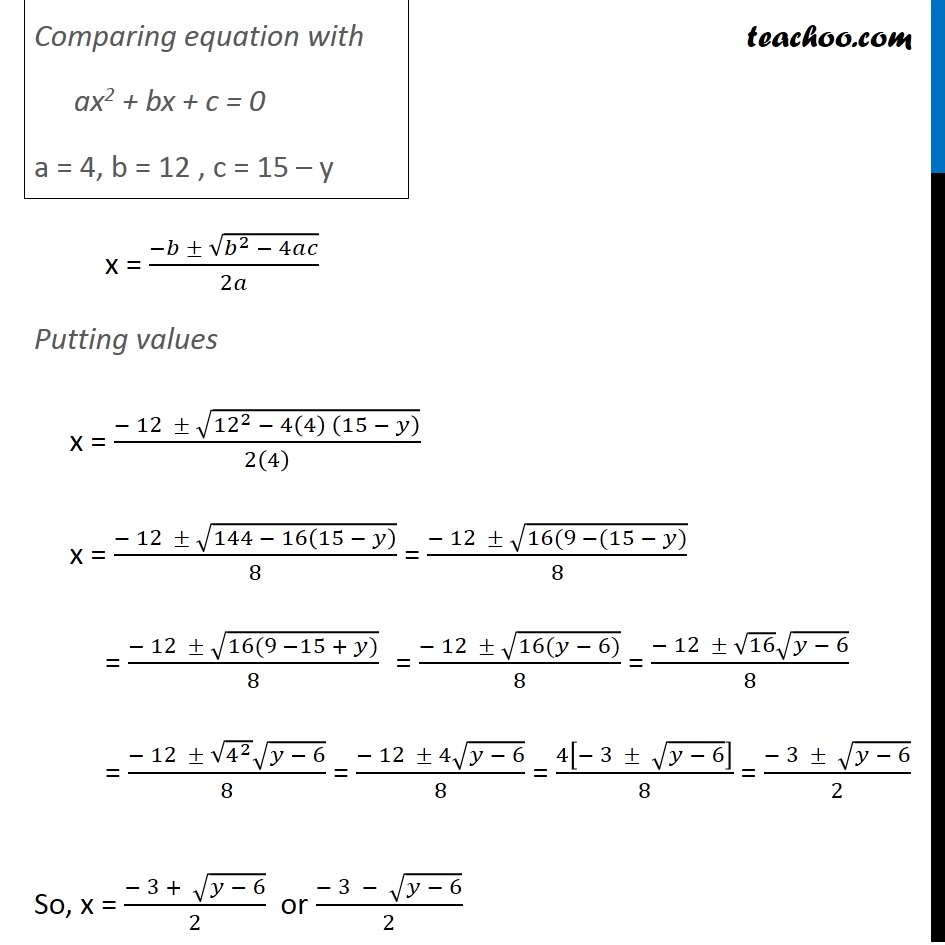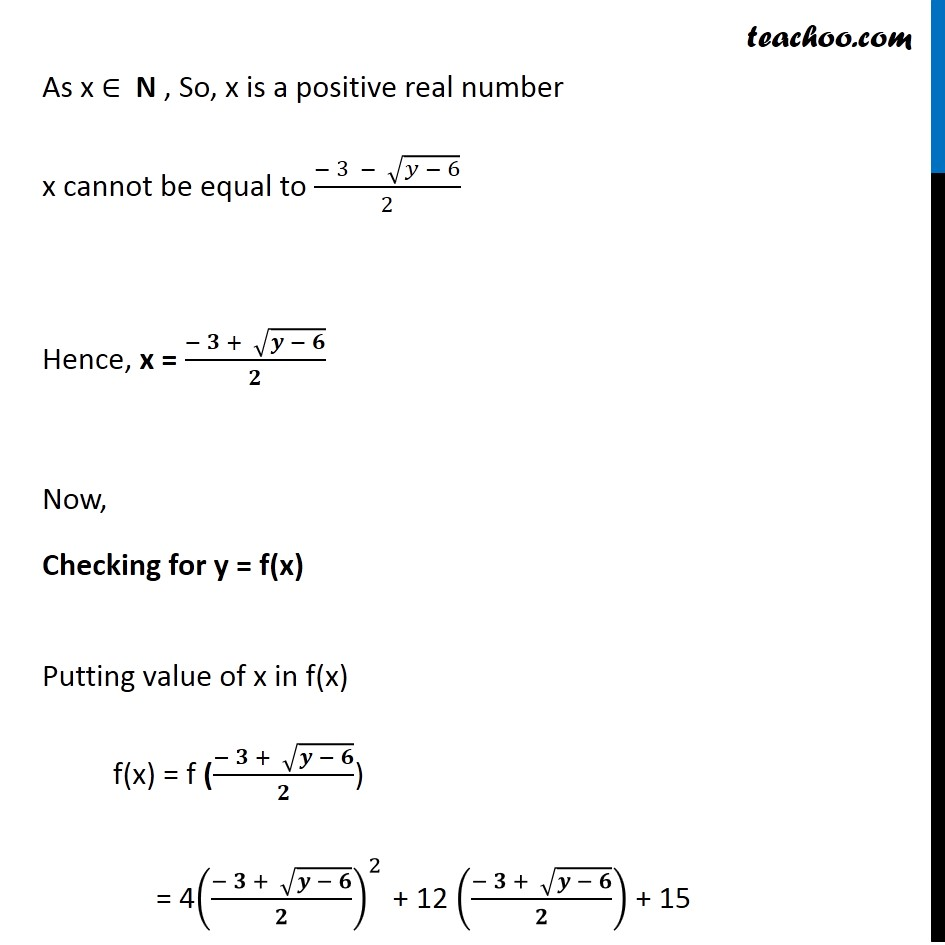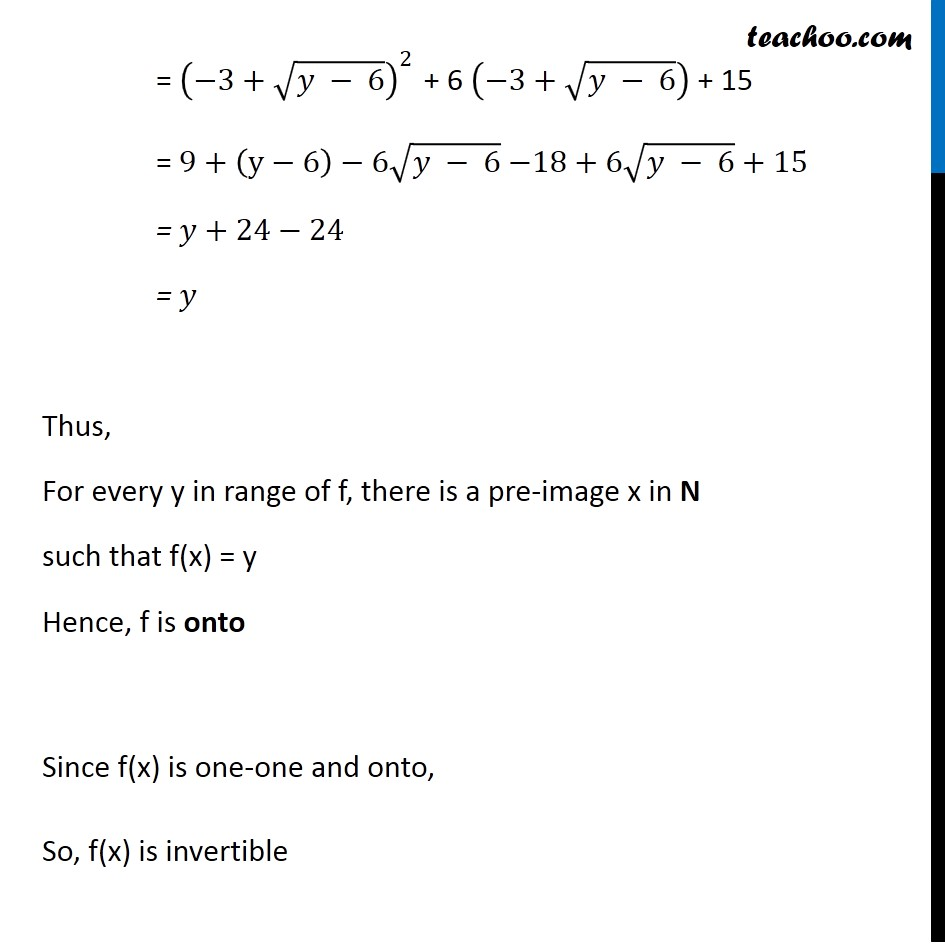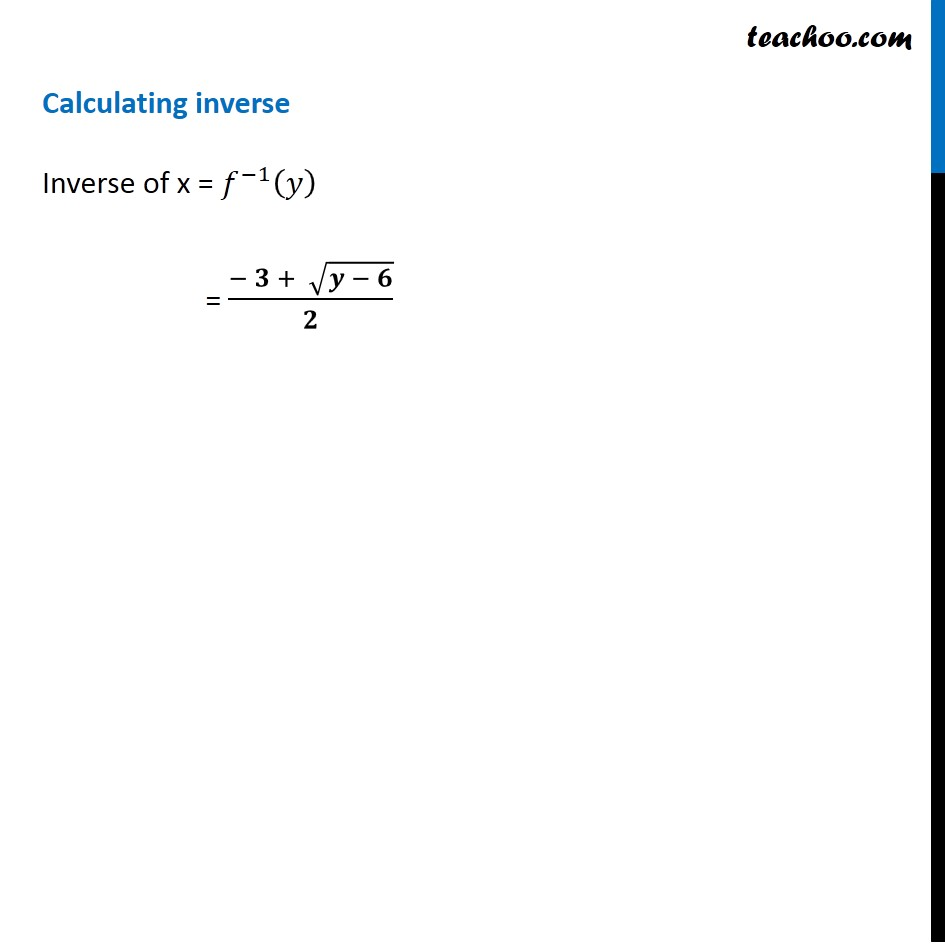Learn in your speed, with individual attention - Teachoo Maths 1-on-1 Class

### Transcript

Question 8 (Method 1) Let f : N → R be a function defined as f (x) = 4x2 + 12x + 15. Show that f : N→ S, where, S is the range of f, is invertible. Find the inverse of f. f(x) = 4x2 + 12x + 15 Step 1: Let f(x) = y y = 4x2 + 12x + 15 0 = 4x2 + 12x + 15 – y 4x2 + 12x + 15 – y = 0 4x2 + 12x + (15 – y) = 0 Rough Checking inverse of f:X → Y Step 1: Calculate g: Y → X Step 2: Prove gof = IX Step 3: Prove fog = IY g is the inverse of f Comparing equation with ax2 + bx + c = 0 a = 4, b = 12 , c = 15 – y x = (−𝑏 ± √(𝑏^2 − 4𝑎𝑐))/2𝑎 Putting values x = (− 12 ± √(〖12〗^2 − 4(4) (15 − 𝑦) ))/2(4) x = (− 12 ± √(144 − 16(15 − 𝑦) ))/8 = (− 12 ± √(16(9 −(15 − 𝑦)))/8 = (− 12 ± √(16(9 −15 + 𝑦)))/8 = (− 12 ± √(16(𝑦 − 6)))/8 = (− 12 ± √16 √(𝑦 − 6))/8 = (− 12 ± √(4^2 ) √(𝑦 − 6))/8 = (− 12 ± 4√(𝑦 − 6))/8 = 4[− 3 ± √(𝑦 − 6)]/8 = (− 3 ± √(𝑦 − 6))/2 So, x = (− 3 + √(𝑦 − 6))/2 or (− 3 − √(𝑦 − 6))/2 As x ∈ N , So, x is a positive real number x cannot be equal to (− 3 − √(𝑦 − 6))/2 Hence, x = (− 𝟑 + √(𝒚 − 𝟔))/𝟐 Let g(y) = (− 3 + √(𝑦 − 6))/2 where g: S → N Rough Checking inverse of f:X → Y Step 1: Calculate g: Y → X Step 2: Prove gof = IX Step 3: Prove fog = IY g is the inverse of f Step 2: gof = g(f(x)) = g(4x2 + 12x + 15) = (−3 + √(4𝑥^2 + 12𝑥 + 15 − 6))/2 = (−3 + √(4𝑥^2 +12𝑥 + 9))/2 = (−3 + √(〖(2𝑥)〗^2+ 3^2 +2(2𝑥) ×3))/2 = (−3 + √((2𝑥 + 3)^2 ))/2 = (−3 + 2𝑥 +3)/2 = 2𝑥/2 = x Hence, gof = x = IN Rough Checking inverse of f:X → Y Step 1: Calculate g: Y → X Step 2: Prove gof = IX Step 3: Prove fog = IY g is the inverse of f Step 3: fog = f(g(x)) = f((− 3 + √(𝑦 − 6))/2) = 4((− 3 + √(𝑦 − 6))/2)^2 + 12((− 3 + √(𝑦 − 6))/2) + 15 = 4(−3 + √(𝑦 − 6))^2/4 + 6(−3 + √(𝑦 −6)) + 15 = (−3 + √(𝑦 −6))^2– 18 + 6√(𝑦 −6) + 15 = (–3)2 + (√(𝑦 −6))^2– 6√(𝑦 −6) – 18 + 6√((6 + 𝑦) ) + 15 = 9 + y – 6 – 18 + 15 = y Hence, fog = y = IS Since, gof = IN & fog = IS f is invertible, and inverse of f = g(y) = (− 𝟑 + √(𝒚 − 𝟔))/𝟐 Question 8 (Method 2) Let f : N → R be a function defined as f (x) = 4x2 + 12x + 15. Show that f : N→ S, where, S is the range of f, is invertible. Find the inverse of f. f(x) = 4x2 + 12x + 15 f is invertible if it is one-one and onto Checking one-one f (x1) = 4(x1)2 + 12x1 + 15 f (x2) = 4(x2)2 + 12x2 + 15 Putting f(x1) = f (x2) 4(x1)2 + 12x1 + 15 = 4(x2)2 + 12x2 + 15 Rough One-one Steps: 1. Calculate f(x1) 2. Calculate f(x2) 3. Putting f(x1) = f(x2) we have to prove x1 = x2 4(x1)2 – 4(x2)2 + 12x1 – 12x2 = 15 – 15 4(x1)2 – 4(x2)2 + 12x1 – 12x2 = 0 4[(x1)2 – (x2)2 ]+ 12[x1 – x2] = 0 4[(x1 – x2) (x1 + x2) ]+ 12[x1 – x2] = 0 4(x1 – x2) [(x1 + x2) + 3] = 0 (x1 – x2) [x1 + x2 + 3] = 0/4 (x1 – x2) [x1 + x2 + 3] = 0 (x1 – x2) = 0 ∴ x1 = x2 (x1 + x2 + 3) = 0 x1 = – x2 – 3 Since f: N → S So x ∈ N i.e. x is always positive, Hence x1 = – x2 – 3 is not true Hence, if f (x1) = f (x2) , then x1 = x2 ∴ f is one-one Check onto f(x) = 4x2 + 12x + 15 Let f(x) = y such that y ∈ S Putting in equation y = 4x2 + 12x + 15 0 = 4x2 + 12x + 15 – y 4x2 + 12x + 15 – y = 0 4x2 + 12x + (15 – y) = 0 Comparing equation with ax2 + bx + c = 0 a = 4, b = 12 , c = 15 – y x = (−𝑏 ± √(𝑏^2 − 4𝑎𝑐))/2𝑎 Putting values x = (− 12 ± √(〖12〗^2 − 4(4) (15 − 𝑦) ))/2(4) x = (− 12 ± √(144 − 16(15 − 𝑦) ))/8 = (− 12 ± √(16(9 −(15 − 𝑦)))/8 = (− 12 ± √(16(9 −15 + 𝑦)))/8 = (− 12 ± √(16(𝑦 − 6)))/8 = (− 12 ± √16 √(𝑦 − 6))/8 = (− 12 ± √(4^2 ) √(𝑦 − 6))/8 = (− 12 ± 4√(𝑦 − 6))/8 = 4[− 3 ± √(𝑦 − 6)]/8 = (− 3 ± √(𝑦 − 6))/2 So, x = (− 3 + √(𝑦 − 6))/2 or (− 3 − √(𝑦 − 6))/2 As x ∈ N , So, x is a positive real number x cannot be equal to (− 3 − √(𝑦 − 6))/2 Hence, x = (− 𝟑 + √(𝒚 − 𝟔))/𝟐 Now, Checking for y = f(x) Putting value of x in f(x) f(x) = f ((− 𝟑 + √(𝒚 − 𝟔))/𝟐) = 4((− 𝟑 + √(𝒚 − 𝟔))/𝟐)^2 + 12 ((− 𝟑 + √(𝒚 − 𝟔))/𝟐) + 15 = (−3+√(𝑦 − 6))^2 + 6 (−3+√(𝑦 − 6)) + 15 = 9+(y−6)−6√(𝑦 − 6) −18+6√(𝑦 − 6)+15 = 𝑦+24−24 = 𝑦 Thus, For every y in range of f, there is a pre-image x in N such that f(x) = y Hence, f is onto Since f(x) is one-one and onto, So, f(x) is invertible Calculating inverse Inverse of x = 𝑓^(−1) (𝑦) = (− 𝟑 + √(𝒚 − 𝟔))/𝟐